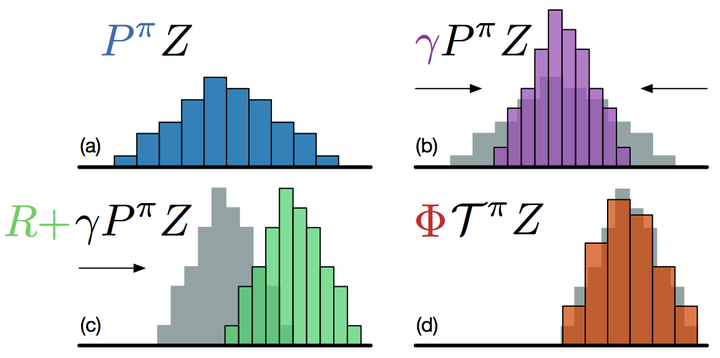# A distributional perspective on reinforcement learningDistributional Bellman equation followed by categorical projection.

### Abstract

In this paper we argue for the fundamental importance of the value distribution: the distribution of the random return received by a reinforcement learning agent. This is in contrast to the common approach to reinforcement learning which models the expectation of this return, or value. Although there is an established body of literature studying the value distribution, thus far it has always been used for a specific purpose such as implementing risk-aware behaviour. We begin with theoretical results in both the policy evaluation and control settings, exposing a significant distributional instability in the latter. We then use the distributional perspective to design a new algorithm which applies Bellman's equation to the learning of approximate value distributions. We evaluate our algorithm using the suite of games from the Arcade Learning Environment. We obtain both state-of-the-art results and anecdotal evidence demonstrating the importance of the value distribution in approximate reinforcement learning. Finally, we combine theoretical and empirical evidence to highlight the ways in which the value distribution impacts learning in the approximate setting.

Publication
In International Conference on Machine Learning